# Functional Programming with Kotlin and Arrow Part 2: Categories and Functors

In this functional programming tutorial, you’ll learn what category theory is, see how to apply it to programming, and learn how to make use of Functors with Arrow.

4.8/5 9 Ratings

#### Version

• Kotlin 1.3, Other, IntelliJ IDEA

When encountering Functional Programming (FP) for the first time, you may ask yourself: “So what?

As in the previous tutorial of this series, the main challenge is to understand abstract concepts, and then apply them to real use cases.

This is especially true when you study one of the most abstract branches of math: category theory. This is an abstract topic, and it’s all about composition, which you might recall are the two superpowers of the FP hero.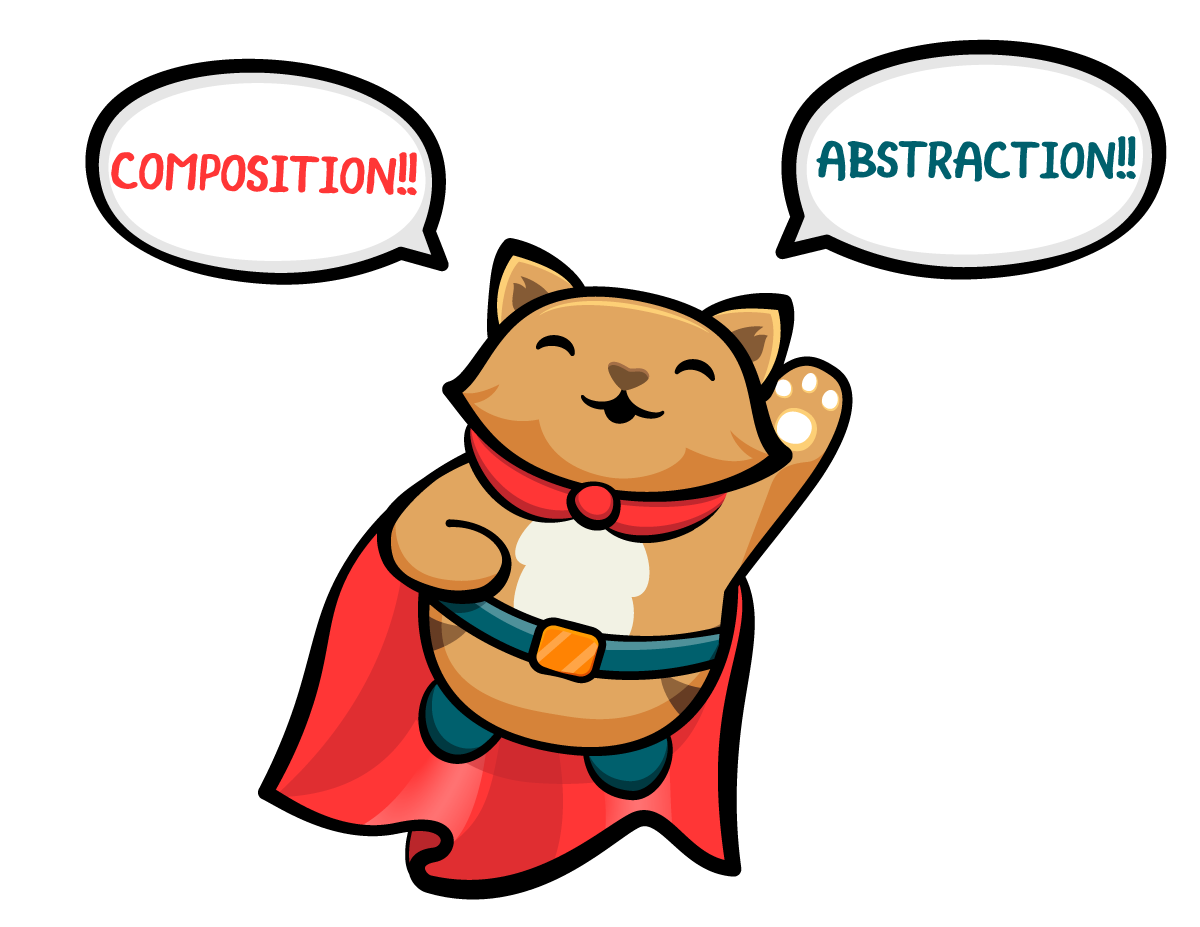Simplifying this theory through a practical approach is also the goal for this tutorial, where you’ll learn:

• What category theory is.
• How to apply it to programming.
• Where the Arrow name came from.
• What a Functor is and how it can be useful.
• The type constructor and the Kind type.
• What a typeclass is.
• How to use List and Maybe as functors

Again, you have a lot to get through, so let’s get started :]

Note: This tutorial series has been inspired by the wonderful Bartosz Milewski’s Category Theory for Programmers course.

## Getting Started

You’ll see two projects in there, one containing the starter project, and another with the final code. Open the starter project in IntelliJ IDEA, where you’ll see a Gradle project with these four modules:

• functions: Contains some simple, pure functions and related tests.
• custom-maybe: You will use this to create your own implementation of the Maybe Functor.
• data-types: Initially empty, you will write code to implement an Arrow-based version of Maybe.
• app: The final app module used to run your Arrow code.

To keep the Arrow-based implementation separated from your own, the custom-maybe module depends only on the functions module. For the same reason, the app module depends on data-types, which itself depends on functions.

The app, custom-maybe and data-types modules also define a dependency on the Arrow framework. This is the start of your second journey from Object Oriented Programming (OOP) to Functional Programming (FP). Again, fasten your seat belts!

## What Is a Category?

Category theory is one of the most abstract branches of mathematics, and it’s an important one because programming is one of its main applications. In this tutorial, you’ll learn the concepts of this theory that can be useful when you apply FP to your code.

What is a category? A category is a bunch of objects, with connections between them called morphisms, plus some rules.

Note: When studying this theory, it’s very important to use the right words. In particular, bunch is appropriate because saying set, for instance, would have implied many rules that wouldn’t be correct in the general case. For instance, a set has in it elements which are unique. A category is more abstract.

You can think of an object like a dot with no properties and no further way to be decomposed. If the object had properties, think of it as a set of them. A morphism is an arrow (rings a bell?) between two objects. Every object of a category must have at least a single morphism — identity — which connects it with itself. As you can see in the following image, different objects can also have a morphism between them, though this is not mandatory: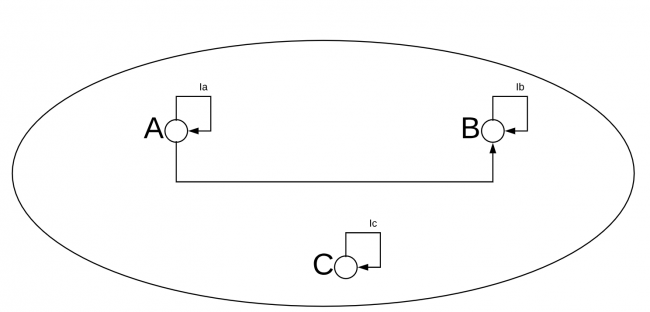Objects and Morphisms

In this image, you can see a category with three objects (A, B and C), their identity morphisms (Ia, Ib and Ic) and a morphism between A and B.

You can have multiple morphisms between two objects A and B. You can even have multiple identities, and therefore multiple morphisms between an object and itself.

### Composition

Objects and morphisms are not enough to define a category: An operation called composition is also required to exist for the morphisms. To understand it, take a look at the following image: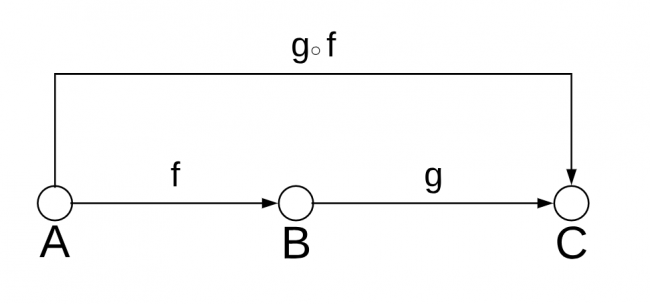Function composition

Here you have a morphism, f, from A to B and a morphism, g, from B to C.

In this case, a category must have another morphism, which you call g after f, from A to C which is the composition of f and g. As in the image, you represent the composition using the small circle . Note that the image doesn’t show the identities, as those are assumed to exist for each object.

Composition has to have the property of associativity which, given three morphisms f, g and h, you can represent as:

h ○ (g ○ f) = (h ○ g) ○ f

Also, in this case, you can use identities in a simple way to replace each morphism.

### The Category of Types and Functions

That “So what?” question might be popping up again. Let’s think about what objects and morphisms can correspond to in programming terms.

What if objects are types and morphisms are functions? You have to prove that this is a category. Given a type T you need an identity, which would be a function that maps values of that type to objects of the same type. In Kotlin you can always write something like:

```fun <T> identity(value: T) = value
```

In this case, you return the same value as the input value. You’ve used Kotlin’s one-line function syntax, in which the return type `T` is inferred by the compiler.

Remember, what matters is the return type, which must be the same as the input parameter’s type. Because of this, you might have infinite identities. However, to prove that this is a category, you only need one.

With that done, you need to prove that you have composition with associativity. You’ve seen composition in the previous tutorial of the series, which Arrow represents like this:

```inline infix fun <B, C, A> ((B) -> C).compose(crossinline f: (A) -> B): (A) -> C = { a: A -> this(f(a)) }
```

If you have a function g of type (B) -> C and a function f of type (A) -> B you can compose them and get a function g ○ f of type (A) -> C.

In the previous snippet, you apply the compose function as an infix operation on g and f, getting a new function as the return value.

### The Associativity of Function Composition

To complete the proof that types and functions are a category, you need to prove that function composition has associativity, which is the following:

```h compose (g compose f) = (h compose g) compose f
```

given that these functions have the following types:

```f: (A) -> B
g: (B) -> C
h: (C) -> D
```

To prove this, keep the previous code and apply the referential transparency you’ve learned about in the previous tutorial to the left and right sides of the equation above. From the left side, you get this:

```h compose (g compose f) = h compose ({ a: A -> g(f(a)) }) = { a: A -> h(g(f(a))) }
```

On the right you get:

```(h compose g) compose f = ({ b: B -> h(g(b)) }) compose f = { a: A -> h(g(f(a))) }
```

These two sides are then identical. You just proved that types and functions create a category!

### Morphisms and Function Types

In the category of types and functions, each object is a type, and each morphism is a function. Given two types, you can have infinite morphisms. The set of all morphisms between two types, which can even be equal in case of identity, has a name: hom-set. A hom-set is a representation of the concept of a function type.

If types and functions create a category, anything you discover to be true in category theory will also be true for types and functions. Identity, composition and associativity are just the tip of the iceberg, and in the next sections, your efforts to prove that types and functions create a category will pay off.

## Mapping Between Categories: Functors

Suppose you have a category C which contains two objects, a and b, and a morphism, f, between them.

You want to define a mapping, which you can call F between a and b and another pair of objects in a different category D, which you’ll call a’ and b’.

You also want F to map morphisms, so it will map f into a morphism f’ in the D category.

Take a look at all of this in this next image, visually:

```a' = F a
b' = F b
f' = F f
```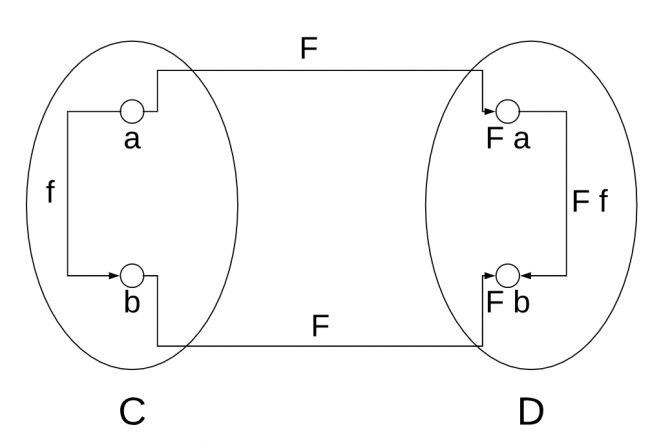Functor definition

For F to be a correct mapping, then if f is a morphism from a to b, `f : (a) -> b`, then the same must be true for their mapped counterparts, so Ff must be a morphism from Fa to Fb:

```F f : (F a) -> F b
```

The F mapping that you’ve defined here is a functor and, thanks to the property above, it maintains the structure of a category. But what is a functor in practice?

### The Maybe Functor

Suppose you have the function `stringToInt` that converts a `String` into the `Int` that it contains. If this is not possible, the function throws an exception.

A function like this is simple and you can find it in the StringToInt.kt file in the functions module:

```val stringToInt: (String) -> Int = Integer::parseInt
```

You need to explicitly type the function to remove the ambiguity with another overload of the `parseInt` method of the `Int` class.

Now, suppose you want to convert an `Int` into the related roman numeral.

To do so, you can use the `intToRoman` function in the IntToRoman.kt file in the same functions module. It uses the `toRoman` function in the Roman.kt file. The type of this function is `(Int?) -> String`.

```val intToRoman: (Int?) -> String = Int?::toRoman
```

It would be nice to compose these two functions in a declarative way. A possible way of doing this is by implementing a functor called `Maybe<T>`.

Navigate to the custom-maybe module, create a CustomMaybe.kt file in the `rw` package and copy this code into the file:

```sealed class Maybe<out T>
class Some<T>(val value: T): Maybe<T>()
object None: Maybe<Nothing>()
```

This is a sealed class with a generic type `T`, which has two possible realizations. The type `Some<T>` represents the case when you can encapsulate a value of type `T`. The second is the case when the result doesn’t exist.

If you think about about the previous `stringToInt` function, this is exactly the type the function should return. Depending on whether the input string can be converted to a valid `Int` value, you might have a result or not.

As a first option, you could rewrite the same function like this in a new file called StringToIntMaybe.kt in the `rw` package, still in the custom-maybe module:

```val stringToIntMaybe: (String) -> Maybe<Int> =
{ s ->
try {
Some(Integer.parseInt(s))
} catch (e: NumberFormatException) {
None
}
}
```

If the input contains something that can be converted to an `Int`, the function will return a `Some` with the value. Otherwise it will return a `None`.

Chaining Operations on Maybe

But what’s the advantage of this?

Remember that your superpowers are abstraction and composition.

What you tried to do here is apply a function to a value of a generic type `T` and get a value as a result or nothing. Then you want to apply other functions like the one which converts the `Int` into roman numerals.

To do that, define the `map` function like this in the CustomMaybe.kt file in the custom-maybe module:

```fun <T, S> Maybe<T>.map(fn: (T) -> S): Maybe<S> = when (this) {
is Some<T> -> Some<S>(fn(this.value))
else -> None
}
```

This is an extension function on the type `Maybe<T>` that has a parameter with a function type, which maps values in `T` into values in `S`. The return type is `Maybe<S>` and it’ll be a `Some<S>` in case the function returns a value, or `None` if it doesn’t. If the receiver of the function is `None`, the result will be `None`.

If you want to compose the function for converting a `String` into `Int` and then get its roman numeral representation, it will be quite easy.

Write and run this code in a file called Main.kt, which you can create in the custom-maybe module:

```fun main() {
stringToIntMaybe("Hello")
.map(intToRoman)
.map { print(it) }
}
```

Run this main function.

You’ll get nothing in output because the input is a `String` that cannot be converted into `Int`, and so the conversion to a roman numeral won’t happen.

Replace the previous code with this:

```fun main() {
stringToIntMaybe("123")
.map(intToRoman)
.map { print(it) }
}
```

If you run this code, you’ll get the following output, which is the roman numeral representation of the number 123.

```CXXIII
```

What you’ve just built in `Maybe<T>` is a functor. It’s a safe way to control side effects.

You’re Maybe<T> is similar to the nullable types that Kotlin gives you for free using the `?` symbol with a type.

### The List Functor

The List interface is one of the most important abstractions when you have to manage collections of data. This is also a functor because it has a `map` function which has this signature:

```public inline fun <T, R> Iterable<T>.map(transform: (T) -> R): List<R>
```

This is an extension function for `Iterable<T>`, and `List` is an `Iterable`. You can see how this definition is very similar to the one you have created for the `Maybe<T>` class.

You can see a pattern here, and this is one of the most powerful properties of category theory.

## Arrow Typeclasses

In OOP, a design pattern is a guideline for a solution to a recurrent problem.

When you find an interface that defines an operation that creates objects of a specific type, you recognize the factory pattern. When you build some UI with nested components, you recognize the usage of the composite pattern.

The same process happens in FP. But how can you define what you have created with `Maybe` and `List`? How can you represent the idea of a Functor in a way that doesn’t depend on the specific type to which it is applied?

Arrow calls this a typeclass, which is a way to describe a reusable coding pattern in FP. This is not intuitive and needs some definitions.

### Type Constructors and Higher Kinds

In the previous examples, you created a `Maybe<T>`. This is a generic type which means that you can define a `Maybe<Int>` or a `Maybe<String>` which are conceptually different types.

You can see the `Maybe<T>` as a way to create different types by changing the value of the type parameter `T`. This is a type constructor,where the input parameter is the generic type, and the result is a specific type.

Arrow represents the idea of type constructors using higher kinds, which are a mechanism introduced in Scala. They represent an abstraction of all type constructors. Arrow defines a `Kind` like this:

```interface Kind<out F, out A>
```

The idea is to consider a type like `Maybe<T>` as a specific `Kind<F, T>` where `F` is `Maybe` and `T` is the usual parameter type.

As such, create a new file Maybe.kt in the `rw` package of the data-types module, and add the following definition:

```import arrow.Kind

class Maybe<T>: Kind<Maybe<T>, T>
```

This definition compiles but it’s not very easy to work with. So, Arrow provides a surrogate type called Of type. In the same file, replace the previous definition of `Maybe` with this:

```// 1
class ForMaybe private constructor()
// 2
typealias MaybeOf<T> = Kind<ForMaybe, T>
// 3
sealed class Maybe<out T> : MaybeOf<T>
class Some<T>(val value: T) : Maybe<T>()
object None : Maybe<Nothing>()
```
1. First, you define the `ForMaybe` type. This is very similar to the definition of `Nothing`. It defines a type but it prevents the creation of instances.
2. Then, you define the type `MaybeOf<T>` as an alias of the type `Kind<ForMaybe, T>`.
3. Finally, you define the type `Maybe<T>` as extension of `MaybeOf<T>` which you recall is a `Kind<ForMaybe, T>`.

You see that `Maybe<T>` is the only implementation of `Kind<ForMaybe, T>` so you can create an extension that gives you a `Maybe<T>` from a `Kind<ForMaybe, T>` with a simple downcast. The name of this extension, by convention, is `fix()`.

Add this code to the same file Maybe.kt:

```fun <T> MaybeOf<T>.fix() = this as Maybe<T>
```

Now you can get the specific implementation `Maybe<T>` from the abstraction `Kind<ForMaybe, T>` simply invoking the `fix()` method.

“So what?!”

What is the advantage of doing this? Since `Maybe<T>` is a `Kind<MaybeOf, T>`, if you define a function for the latter, it will be available to use for the former.

For example, imagine that you define a `map` function with this signature:

```fun <F, A, B> Kind<F, A>.map(f: (A) -> B): Kind<F, B>
```

Since you’ve defined it as extension function of the type `Kind<F, A>`, it’s available for all the `Kind` specializations, including your `Maybe<T>` type.

The way you’ll work with higher kinded types most of the time is in the following pattern:

1. Create an instance of your own type. For example, `Maybe<T>`.
2. Work with this value by using all the functionality available for its `Kind` abstraction.
3. Finally, you can get your own concrete type back by using the `fix` function.

### Arrow Data Types

In the previous paragraph, you had to create the Of type for the Maybe type because Kotlin is missing a way to represent the concept of a type constructor. Fortunately, you don’t have to create all that code because Arrow will do it for you using the @higherkind annotation.

Replace all the code in the Maybe.kt file with the following:

```package rw

import arrow.higherkind

@higherkind
sealed class Maybe<out A> : MaybeOf<A> {
companion object
}

data class Some<T>(val value: T) : Maybe<T>()
object None : Maybe<Nothing>()
```

Note the presence of an empty companion object, which is required since Arrow uses it as the anchor point for some generated code.

It’s important to note that this code won’t compile until you build the related module. That is because the `MaybeOf` type is one that Arrow will generate for you. Go ahead and choose Build Project from the Build menu.

You can check the generated code by looking at the higherkind.rw.Maybe.kt file in the build folder, which you can find following the path in the following picture: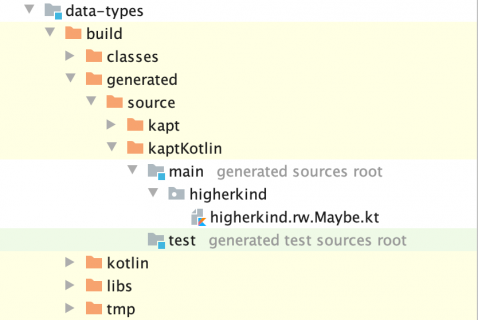Arrow generated code for data types

If you open that file, you’ll see that the generated code is practically the same as what you created earlier by hand, `fix()` method included:

```class ForMaybe private constructor() { companion object }
typealias MaybeOf<A> = arrow.Kind<ForMaybe, A>

@Suppress("UNCHECKED_CAST", "NOTHING_TO_INLINE")
inline fun <A> MaybeOf<A>.fix(): Maybe<A> =
this as Maybe<A>
```

Using the `@higherkind` annotation, you can create what Arrow calls Data Types.

### Functors in Arrow

The Maybe data type you just created is not a functor yet. You need to implement a Type class through what Arrow calls an extension. Arrow makes this easy.

Create a new file MaybeFunctor.kt in the rw package of the app module, and add the following code:

```import arrow.Kind
import arrow.extension
import arrow.typeclasses.Functor

// 1
@extension
// 2
interface MaybeFunctor : Functor<ForMaybe> {
// 3
override fun <A, B> Kind<ForMaybe, A>.map(fn: (A) -> B): Kind<ForMaybe, B> {
// 4
val maybe = this.fix()
return when (maybe) {
is Some<A> -> Some<B>(fn(maybe.value))
else -> None
}
}

companion object
}
```

This code contains several interesting points:

1. The @extension annotation triggers the Arrow code generation on the specific type class.
2. In this case, you create an implementation of the `Functor<T>` interface Arrow provides. If you look at its code (not shown here for brevity) you can see that it defines `map` and other functions based on that. You can see, for instance, the `lift` function, which converts `map()`, receives a function as its parameter into another function that receives the value to which the function should be applied. You’ll see more of that in a future tutorial.
3. Then you define the required `map` function in a similar way to what you already did in the custom implementation, but with a fundamental property: It’s defined on `Kind<ForMaybe, B>`.
4. Thanks to the previous point, you can use the `fix()` function to get your concrete data type to work with in your implementation of `map()`.

The `map()` function alone doesn’t make your data type a functor. As you’ve seen in the first part of the tutorial, the other fundamental properties of identity, composition and associativity must be true.

As a first step, copy the StringToIntMaybe.kt file from the custom-maybe module to the app module. This looks like a repetition of the code, but the `Maybe` types that you use in the two modules are not the same.

Now, create a Main.kt file in the `rw` package of the app module, and add the following code:

```fun main() {
stringToIntMaybe("Hello")
.map(intToRoman)
.map { print(it) }
stringToIntMaybe("123")
.map(intToRoman)
.map { print(it) }
}
```

When you run this code, you’ll see that only the second usage produces output, the expected roman numberal. Your `MaybeFunctor` from Arrow is working as expected?

## Where to Go From Here?

In this tutorial, you created your own custom `Maybe` functor as well as a `Maybe` data type using the Arrow framework. You understood what a type constructor is and how Arrow abstracts it using `Kind`. Then you created an extension of the `Functor` typeclass.

You’ve done this after an important theoretical introduction to what a Category is, what its properties are and how a Functor fits into all of this.

With all that behind you, you’re still at the beginning of the journey into Functional Programming with Arrow.

We hope you enjoyed this tutorial, and if you have any questions or comments, please join the forum discussion below!

4.8/5

9 ratings